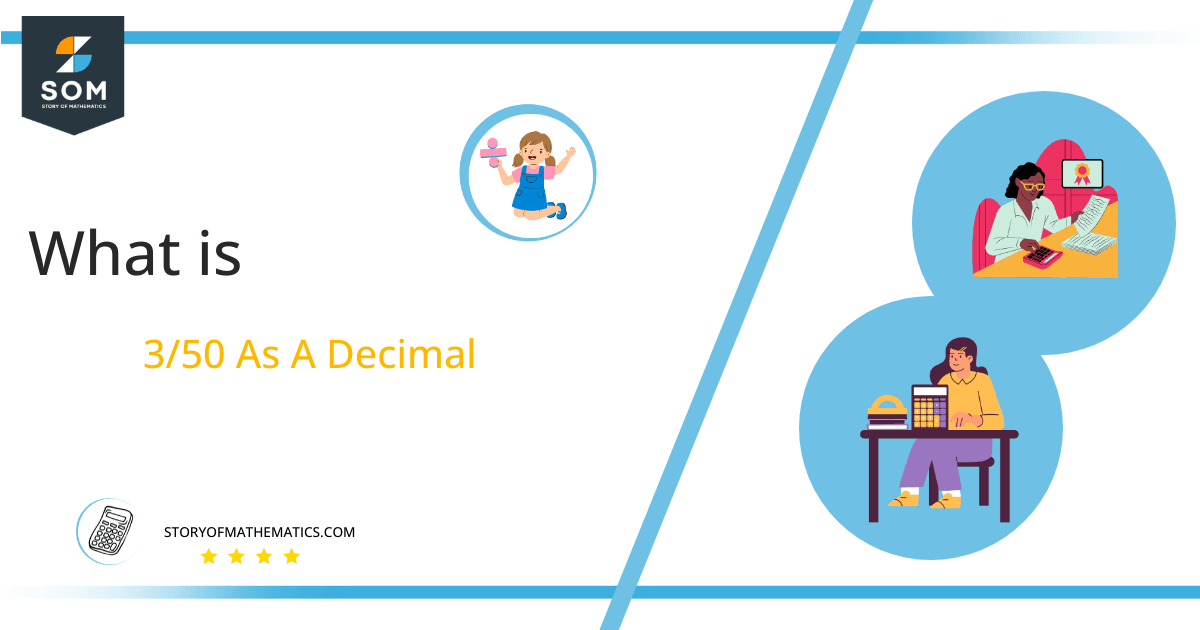# What Is 3/50 as a Decimal + Solution With Free Steps

The fraction 3/50 as a decimal is equal to 0.06.

The fraction is shown in p/q form, with the division line between p and q. The fraction’s p is referred to as the numerator, while its q is referred to as the denominator. We can convert fractions to decimal values by applying the mathematical operation known as division.

Here, we are interested more in the types of division that results in a Decimal value, as this can be expressed as a Fraction. We see fractions as a way of showing two numbers having the operation of Division between them that result in a value that lies between two Integers.Now, we introduce the method used to solve said fraction to decimal conversion, called Long Division which we will discuss in detail moving forward. So, let’s go through the Solution of fraction 3/50.

## Solution

First, we convert the fraction components i.e., the numerator and the denominator, and transform them into the division constituents i.e., the Dividend and the Divisor respectively.

This can be seen done as follows:

Dividend = 3

Divisor = 50

Now, we introduce the most important quantity in our process of division, this is the Quotient. The value represents the Solution to our division, and can be expressed as having the following relationship with the Division constituents:

Quotient = Dividend $\div$ Divisor = 3 $\div$ 50

This is when we go through the Long Division solution to our problem.Figure 1

### 3/50 Long Division Method

We start solving a problem using the Long Division Method by first taking apart the division’s components and comparing them. As we have 3, and 50 we can see how 3 is Smaller than 50, and to solve this division we require that 3 be Bigger than 50.

This is done by multiplying the dividend by 10 and checking whether it is bigger than the divisor or not. And if it is then we calculate the Multiple of the divisor which is closest to the dividend and subtract it from the Dividend. This produces the Remainder which we then use as the dividend later.

Now, we begin solving for our dividend 3, which after getting multiplied by 10 becomes 30.

Still, the dividend is less than the divisor, so we will multiply it by 10 again. For that, we have to add the zero in the quotient. So, by multiplying the dividend by 10 twice in the same step and by adding zero after the decimal point in the quotient, we now have a dividend of 300.

We take this 300 and divide it by 50, this can be seen done as follows:

300 $\div$ 50 = 6

Where:

50 x 6 = 300

This will lead to the generation of a Remainder equal to 300 – 300 = 0.

So, we have a Quotient generated after combining the three pieces of it as 0.06 = z, with a Remainder equal to 0.Images/mathematical drawings are created with GeoGebra.